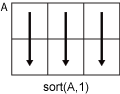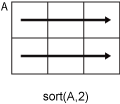# sort

Sort array elements

## Syntax

``B = sort(A)``
``B = sort(A,dim)``
``B = sort(___,direction)``
``B = sort(___,Name,Value)``
``````[B,I] = sort(___)``````

## Description

example

````B = sort(A)` sorts the elements of `A` in ascending order.If `A` is a vector, then `sort(A)` sorts the vector elements.If `A` is a matrix, then `sort(A)` treats the columns of `A` as vectors and sorts each column.If `A` is a multidimensional array, then `sort(A)` operates along the first array dimension whose size does not equal 1, treating the elements as vectors.```

example

````B = sort(A,dim)` returns the sorted elements of `A` along dimension `dim`. For example, if `A` is a matrix, then `sort(A,2)` sorts the elements of each row.```

example

````B = sort(___,direction)` returns sorted elements of `A` in the order specified by `direction` using any of the previous syntaxes. `'ascend'` indicates ascending order (the default) and `'descend'` indicates descending order.```

example

````B = sort(___,Name,Value)` specifies additional parameters for sorting. For example, `sort(A,'ComparisonMethod','abs')` sorts the elements of `A` by magnitude.```

example

``````[B,I] = sort(___)``` also returns a collection of index vectors for any of the previous syntaxes. `I` is the same size as `A` and describes the arrangement of the elements of `A` into `B` along the sorted dimension. For example, if `A` is a vector, then `B = A(I)`.```

## Examples

collapse all

Create a row vector and sort its elements in ascending order.

```A = [9 0 -7 5 3 8 -10 4 2]; B = sort(A)```
```B = 1×9 -10 -7 0 2 3 4 5 8 9 ```

Create a matrix and sort each of its rows in ascending order.

`A = [3 6 5; 7 -2 4; 1 0 -9]`
```A = 3×3 3 6 5 7 -2 4 1 0 -9 ```
`B = sort(A,2)`
```B = 3×3 3 5 6 -2 4 7 -9 0 1 ```

Create a matrix and sort its columns in descending order.

`A = [10 -12 4 8; 6 -9 8 0; 2 3 11 -2; 1 1 9 3]`
```A = 4×4 10 -12 4 8 6 -9 8 0 2 3 11 -2 1 1 9 3 ```
`B = sort(A,'descend')`
```B = 4×4 10 3 11 8 6 1 9 3 2 -9 8 0 1 -12 4 -2 ```

Starting in R2017a, you can create string arrays using double quotes, and sort them using the `sort` function. Sort strings in each column of a string array according to Unicode® dictionary order.

```A = ["Santos","Burns"; ... "Jones","Morita"; ... "Petrov","Adams"]; B = sort(A)```
```B = 3x2 string "Jones" "Adams" "Petrov" "Burns" "Santos" "Morita" ```

Sort the strings in each row.

`B = sort(A,2)`
```B = 3x2 string "Burns" "Santos" "Jones" "Morita" "Adams" "Petrov" ```

Create an array of `datetime` values and sort them in ascending order, that is, from the earliest to the latest calendar date.

```ds = {'2012-12-22';'2063-04-05';'1992-01-12'}; A = datetime(ds,'Format','yyyy-MM-dd')```
```A = 3x1 datetime 2012-12-22 2063-04-05 1992-01-12 ```
`[B,I] = sort(A)`
```B = 3x1 datetime 1992-01-12 2012-12-22 2063-04-05 ```
```I = 3×1 3 1 2 ```

`B` lists the sorted dates and `I` contains the corresponding indices of `A`.

Access the sorted elements from the original array directly by using the index array `I`.

`A(I)`
```ans = 3x1 datetime 1992-01-12 2012-12-22 2063-04-05 ```

Create two row vectors that contain related data in the corresponding elements.

```X = [3 6 4 2 1 5]; Y = ["yellow" "purple" "green" "orange" "red" "blue"];```

First sort the vector `X`, then sort the vector `Y` in the same order as `X`.

`[Xsorted,I] = sort(X)`
```Xsorted = 1×6 1 2 3 4 5 6 ```
```I = 1×6 5 4 1 3 6 2 ```
`Ysorted = Y(I)`
```Ysorted = 1x6 string "red" "orange" "yellow" "green" "blue" "purple" ```

Create a 2-by-2-by-2 array and sort its elements in ascending order along the third dimension.

```A(:,:,1) = [2 3; 1 6]; A(:,:,2) = [-1 9; 0 12]; A```
```A = A(:,:,1) = 2 3 1 6 A(:,:,2) = -1 9 0 12 ```
`B = sort(A,3)`
```B = B(:,:,1) = -1 3 0 6 B(:,:,2) = 2 9 1 12 ```

Use `A(:)`, the column representation of `A`, to sort all of the elements of `A`.

`B = sort(A(:))`
```B = 8×1 -1 0 1 2 3 6 9 12 ```

Sort the elements of a complex vector by their real parts. By default, the `sort` function sorts complex values by their magnitude, and breaks ties using phase angles. Specify the value of `'ComparisonMethod'` as `'real'` to instead sort complex values by their real parts. For elements with equal real parts, `sort` breaks the tie based on their imaginary parts.

```A = [1+2i 3+1i 1i 0 -1i]; B = sort(A,'ComparisonMethod','real')```
```B = 1×5 complex 0.0000 - 1.0000i 0.0000 + 0.0000i 0.0000 + 1.0000i 1.0000 + 2.0000i 3.0000 + 1.0000i ```

## Input Arguments

collapse all

Input array, specified as a vector, matrix, or multidimensional array.

• If `A` is a scalar, then `sort(A)` returns `A`.

• If `A` is complex, then by default, `sort` sorts the elements by magnitude. If more than one element has equal magnitude, then the elements are sorted by phase angle on the interval (−π, π].

• If `A` is a cell array of character vectors or a string array, then `sort(A)` sorts the elements according to the code order for the UTF-16 character encoding scheme. The sort is case-sensitive. For more information on sorting character and string arrays, see Sort Order for Character and String Arrays.

• If `A` is a string array, then `sort` reorders the elements of the array, but does not reorder characters within the strings.

• If `A` is a categorical array, then the sorting order is based on the category order returned by `categories(A)`.

Data Types: `double` | `single` | `int8` | `int16` | `int32` | `int64` | `uint8` | `uint16` | `uint32` | `uint64` | `logical` | `char` | `string` | `cell` | `categorical` | `datetime` | `duration`
Complex Number Support: Yes

Dimension to operate along, specified as a positive integer scalar. If no value is specified, then the default is the first array dimension whose size does not equal 1.

• Consider a matrix `A`. `sort(A,1)` sorts the elements in the columns of `A`.• `sort(A,2)` sorts the elements in the rows of `A`.`sort` returns `A` if `dim` is greater than `ndims(A)`. `dim` is not supported when `A` is a cell array, that is, `sort` only operates along the first array dimension whose size does not equal 1.

Data Types: `double` | `single` | `int8` | `int16` | `int32` | `int64` | `uint8` | `uint16` | `uint32` | `uint64`

Sorting direction, specified as `'ascend'` or `'descend'`. `direction` is not supported when `A` is a cell array, that is, `sort` only sorts in ascending order.

### Name-Value Arguments

Specify optional pairs of arguments as `Name1=Value1,...,NameN=ValueN`, where `Name` is the argument name and `Value` is the corresponding value. Name-value arguments must appear after other arguments, but the order of the pairs does not matter.

Before R2021a, use commas to separate each name and value, and enclose `Name` in quotes.

Example: `sort(A,'MissingPlacement','last')`

Placement of missing values (`NaN`, `NaT`, `<undefined>`, and `missing`) specified as the comma-separated pair consisting of `'MissingPlacement'` and one of the following:

• `'auto'` — Missing elements are placed last for ascending order and first for descending order.

• `'first'` — Missing elements are placed first.

• `'last'` — Missing elements are placed last.

Element comparison method, specified as the comma-separated pair consisting of `'ComparisonMethod'` and one of the following:

• `'auto'` — Sort `A` by `real(A)` when `A` is real, and sort by `abs(A)` when `A` is complex.

• `'real'` — Sort `A` by `real(A)` when `A` is real or complex. If `A` has elements with equal real parts, then use `imag(A)` to break ties.

• `'abs'` — Sort `A` by `abs(A)` when `A` is real or complex. If `A` has elements with equal magnitude, then use `angle(A)` in the interval (-π,π] to break ties.

## Output Arguments

collapse all

Sorted array, returned as a vector, matrix, or multidimensional array. `B` is the same size and type as `A`.

Data Types: `double` | `single` | `int8` | `int16` | `int32` | `int64` | `uint8` | `uint16` | `uint32` | `uint64` | `logical` | `char` | `string` | `cell` | `categorical` | `datetime` | `duration`

Sort index, returned as a vector, matrix, or multidimensional array. `I` is the same size as `A`. The index vectors are oriented along the same dimension that `sort` operates on. For example, if `A` is a 2-by-3 matrix, then ```[B,I] = sort(A,2)``` sorts the elements in each row of `A`. The output `I` is a collection of 1-by-3 row index vectors describing the rearrangement of each row of `A`.

When the input contains repeated values, the sort index preserves the original order in the input, regardless of sort direction. For example, if `A = [1 2 1 2]`, then ```[Ba,Ia] = sort(A,'ascend')``` returns the sort index ```Ia = [1 3 2 4]``` and `[Bd,Id] = sort(A,'descend')` returns the sort index `Id = [2 4 1 3]`.

collapse all

### Sort Order for Character and String Arrays

MATLAB® stores characters as Unicode® using the UTF-16 character encoding scheme. Character and string arrays are sorted according to the UTF-16 code point order. For the characters that are also the ASCII characters, this order means that uppercase letters come before lowercase letters. Digits and some punctuation also come before letters.

## Tips

• The `sortrows` function provides additional flexibility for subsorting over multiple columns of matrix or table inputs.

• The `sort` function and the relational operators use different orderings for complex numbers. For more information, see Relational Operations.

## Version History

Introduced before R2006a